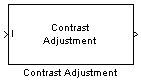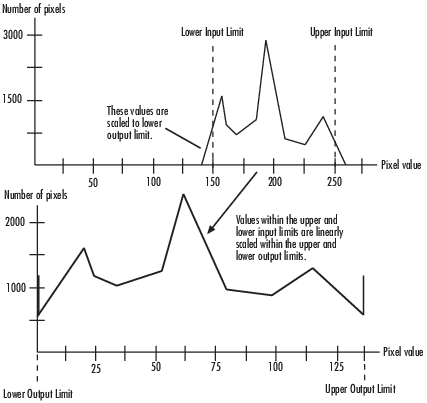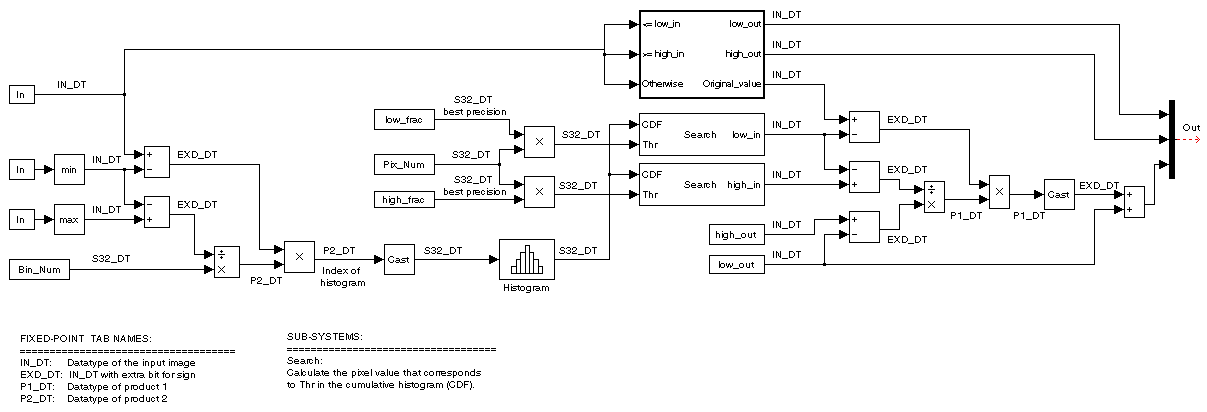Adjust image contrast by linearly scaling pixel values

## Library

Analysis & Enhancement

`visionanalysis`

•## Description

The Contrast Adjustment block adjusts the contrast of an image by linearly scaling the pixel values between upper and lower limits. Pixel values that are above or below this range are saturated to the upper or lower limit value, respectively.Mathematically, the contrast adjustment operation is described by the following equation, where the input limits are [low_in high_in] and the output limits are [low_out high_out]:

`$Output=\left\{\begin{array}{c}low_out\begin{array}{cc},& Input\le low_in\end{array}\\ low_out+\left(Input-low_in\right)\frac{high_out-low_out}{high_in-low_in}\begin{array}{cc},& low_in`
PortInput/OutputSupported Data TypesComplex Values Supported

I

Vector or matrix of intensity values

• Double-precision floating point

• Single-precision floating point

• Fixed point

• 8-, 16-, and 32-bit signed integer

• 8-, 16-, and 32-bit unsigned integer

No

Output

Scalar, vector, or matrix of intensity values or a scalar, vector, or matrix that represents one plane of the RGB video stream

Same as I port

No

### Specifying upper and lower limits

Use the Adjust pixel values from and Adjust pixel values to parameters to specify the upper and lower input and output limits. All options are described below.

Input limits

Use the Adjust pixel values from parameter to specify the upper and lower input limits.

 If you select `Full input data range [min max]`, uses the minimum input value as the lower input limit and the maximum input value as the upper input limit.
 If you select `User-defined`, the Range [low high] parameter associated with this option appears. Enter a two-element vector of scalar values, where the first element corresponds to the lower input limit and the second element corresponds to the upper input limit.
 If you select ```Range determined by saturating outlier pixels```, the Percentage of pixels to saturate [low high] (in %), Specify number of histogram bins (used to calculate the range when outliers are eliminated), and Number of histogram bins parameters appear on the block. The block uses these parameter values to calculate the input limits in this three-step process:
1. Find the minimum and maximum input values, [min_in max_in].

2. Scale the pixel values from [min_in max_in] to [0 num_bins-1], where num_bins is the scalar value you specify in the Number of histogram bins parameter. This parameter always displays the value used by the block. Then the block calculates the histogram of the scaled input. For additional information about histograms, see the 2D-Histogram block reference page.

3. Find the lower input limit such that the percentage of pixels with values smaller than the lower limit is at most the value of the first element of the Percentage of pixels to saturate [low high] (in %) parameter. Similarly, find the upper input limit such that the percentage of pixels with values greater than the upper limit is at least the value of the second element of the parameter.

Output limits

Use the Adjust pixel values to parameter to specify the upper and lower output limits.

 If you select `Full data type range`, the block uses the minimum value of the input data type as the lower output limit and the maximum value of the input data type as the upper out
 If you select `User-defined range`, the Range [low high] parameter appears on the block. Enter a two-element vector of scalar values, where the first element corresponds to the lower output limit and the second element corresponds to the upper output limit.

For INF, -INF and NAN Input Values

If any input pixel value is either `INF` or `-INF`, the Contrast Adjustment block will change the pixel value according to how the parameters are set. The following table shows how the block handles these pixel values.

If Adjust pixel values from parameter is set to...Contrast Adjustment block will:
Full data range [min,max]

Set the entire output image to the lower limit of the Adjust pixel values to parameter setting.

Range determined by saturating outlier pixels
User defined rangeLower and higher limits of the Adjust pixel values to parameter set to `-INF` and `INF `, respectively.

If any input pixel has a `NAN` value, the block maps the pixels with valid numerical values according to the user-specified method. It maps the `NAN` pixels to the lower limit of the Adjust pixels values to parameter.

### Examples

See Adjust the Contrast of Intensity Images in the Computer Vision Toolbox™ User's Guide.

### Fixed-Point Data Types

The following diagram shows the data types used in the Contrast Adjustment block for fixed-point signals:## Parameters

Specify how to enter the upper and lower input limits. Your choices are `Full input data range [min max]`, `User-defined`, and `Range determined by saturating outlier pixels`.

Range [low high]

Enter a two-element vector of scalar values. The first element corresponds to the lower input limit, and the second element corresponds to the upper input limit. This parameter is visible if, for the Adjust pixel values from parameter, you select `User-defined`.

Percentage of pixels to saturate [low high] (in %)

Enter a two-element vector. The block calculates the lower input limit such that the percentage of pixels with values smaller than the lower limit is at most the value of the first element. It calculates the upper input limit similarly. This parameter is visible if, for the Adjust pixel values from parameter, you select ```Range determined by saturating outlier pixels```.

Specify number of histogram bins (used to calculate the range when outliers are eliminated)

Select this check box to change the number of histogram bins. This parameter is editable if, for the Adjust pixel values from parameter, you select ```Range determined by saturating outlier pixels```.

Number of histogram bins

Enter the number of histogram bins to use to calculate the scaled input values. This parameter is available if you select the Specify number of histogram bins (used to calculate the range when outliers are eliminated) check box.

Specify the upper and lower output limits. If you select ```Full data type range```, the block uses the minimum value of the input data type as the lower output limit and the maximum value of the input data type as the upper output limit. If you select ```User-defined range```, the Range [low high] parameter appears on the block.

Range [low high]

Enter a two-element vector of scalar values. The first element corresponds to the lower output limit and the second element corresponds to the upper output limit. This parameter is visible if, for the Adjust pixel values to parameter, you select ```User-defined range```

Rounding mode

Select the rounding mode for fixed-point operations.

Overflow mode

Select the overflow mode for fixed-point operations.

Product 1

The product output type when the block calculates the ratio between the input data range and the number of histogram bins.As shown in the previous figure, the output of the multiplier is placed into the product output data type and scaling. Use this parameter to specify how to designate this product output word and fraction lengths:

When you select `Binary point scaling`, you can enter the word length and the fraction length of the product output, in bits.

When you select `Slope and bias scaling`, you can enter the word length, in bits, and the slope of the product output. The bias of all signals in the Computer Vision Toolbox software is 0.

Product 2

The product output type when the block calculates the bin location of each input value.As shown in the previous figure, the output of the multiplier is placed into the product output data type and scaling. Use this parameter to specify how to designate this product output word and fraction lengths:

When you select `Binary point scaling`, you can enter the word length and the fraction length of the product output, in bits.

When you select `Slope and bias scaling`, you can enter the word length, in bits, and the slope of the product output. The bias of all signals in the Computer Vision Toolbox software is 0.

This parameter is visible if, for the Adjust pixel values from parameter, you select ```Range determined by saturating outlier pixels```.

Lock data type settings against change by the fixed-point tools

Select this parameter to prevent the fixed-point tools from overriding the data types you specify on the block mask. For more information, see `fxptdlg` (Fixed-Point Designer), a reference page on the Fixed-Point Tool in the Simulink® documentation.

 2D-Histogram Computer Vision Toolbox software Histogram Equalization Computer Vision Toolbox software

## Extended Capabilities

### C/C++ Code GenerationGenerate C and C++ code using Simulink® Coder™.

Introduced in R2006b